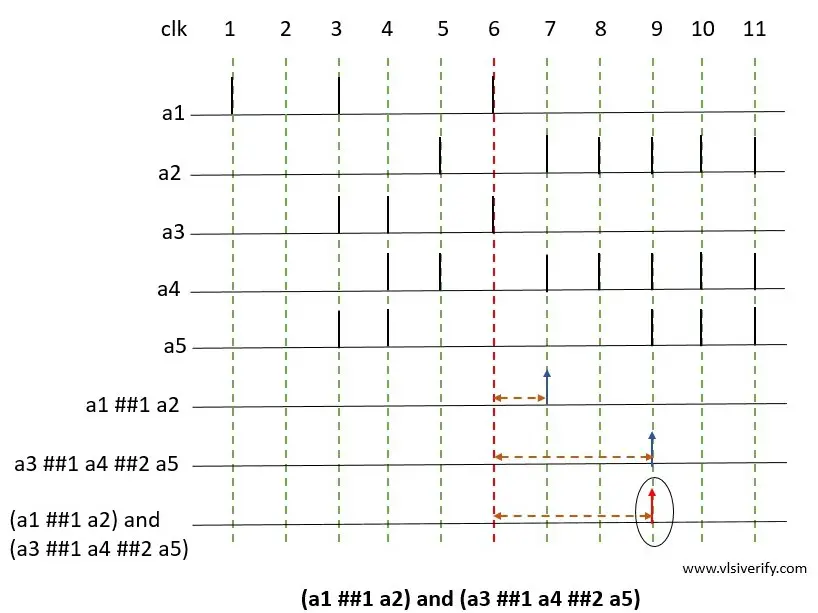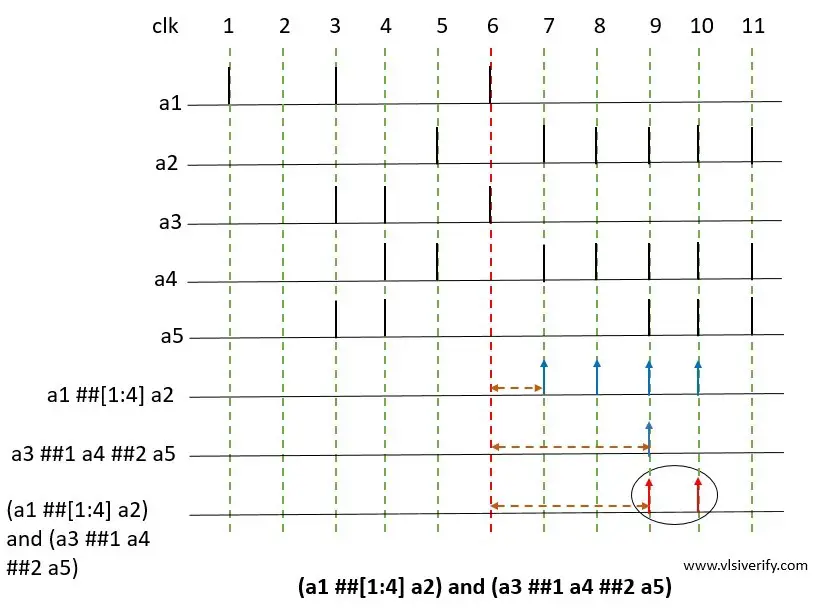Tutorials

# and operator (Conjunction)

The binary and operator are used when two sequence operands are expected to match, but the end time of both operand sequences can be different.

Syntax:

``<seq_exp> and <seq_exp>``

The requirement for and operation match

1. Both operand sequences should start at the same time.
2. Both operands must match. In case one operand matches, it waits for another operand to match.
3. The end time of the resultant or composite sequence is the end time of the operand that finishes last.

## Example for AND of two sequences

``(a1 ##1 a2) and (a3 ##1 a4 ##2 a5)``### Explanation

Let’s consider  a1 ##1 a2 as operand A and a3 ##1 a4 ##2 a5 as operand B.operand B

a1 ##1 a2 (Operand A): The a1 evaluates to true followed by a2 one clock tick matches at clock tick 7

a3 ##1 a4 ##2 a5 (operand B): The operand a3 evaluates to true followed by a4 one clock tick later, followed by a5 two clock ticks later i.e. at clock tick 9.

(a1 ##1 a2) and (a3 ##1 a4 ##2 a5): The resultant sequence is said to be matched when both of the above operand sequence matches. The end times of matches for individual operand sequences A and B are clock ticks 7 and 9 respectively.

The resultant sequence match should follow the conditions like individual operand sequences should start at the same clock tick (clock tick 6) and the end time of the last finishing operand sequence will be the end time of the resultant sequence (clock tick 9).

An important point to note here: The two operand sequences need not be matched at the same number of clock ticks.

## Example for AND of two sequences including a time range

`` (a1 ##[1:4] a2) and (a3 ##1 a4 ##2 a5)``### Explanation

Let’s consider  a1 ##[1:4] a2 as operand A and a3 ##1 a4 ##2 a5 as operand B.operand B

a1 ##[1:4] a2: The first operand a1 evaluates to true followed by a2 which evaluates to true for 1,2,3, or 4 clock ticks later. So, sequence matches at clock ticks 7,8,9, and 10 respectively.

a3 ##1 a4 ##2 a5: The operand a3 evaluates to true followed by a4 one clock tick later, followed by a5 two clock ticks later i.e. at clock tick 9.

(a1 ##1 a2) and (a3 ##1 a4 ##2 a5): The resultant sequence is said to be matched when both of the above operand sequence matches. The end times of matches for individual operand sequences are clock ticks 10 and 9.

The resultant sequence match should follow the conditions like individual operand sequences should start at the same clock tick (clock tick 6) and end time of resultant sequence can have two matches possible at clock tick 9 and 10 since if operand A match with operand B at clock tick 9 and operand A has end time at clock tick 10 as a last finishing operand sequence.

SystemVerilog Assertions